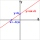# Line + intersection of sets - math problems

#### Number of problems found: 7

• Line intersect segmentDecide whether the line p : x + 2 y - 7 = 0 intersects the line segment given by points A[1, 1] and B[5, 3]
• The triangleThe triangle is given by three vertices: A [0.0] B [-4.2] C [-6.0] Calculate V (intersection of heights), T (center of gravity), O - center of a circle circumscribed
• CoordinatesDetermine the coordinates of the vertices and the content of the parallelogram, the two sides of which lie on the lines 8x + 3y + 1 = 0, 2x + y-1 = 0 and the diagonal on the line 3x + 2y + 3 = 0
• SegmentsLine segments 62 cm and 2.2 dm long we divide into equal parts which lengths in centimeters is expressed integer. How many ways can we divide?
• Perpendicular projectionDetermine the distance of a point B[1, -3] from the perpendicular projection of a point A[3, -2] on a straight line 2 x + y + 1 = 0.
• Construction of trapezoidConstruct a trapezoid if b = 4cm, c = 7cm, d = 4,5cm, v = 3 cm (Procedure, discussion, sketch, analysis, construction)
• Eq2 2Solve following equation with quadratic members and rational function: (x2+1)/(x-4) + (x2-1)/(x+3) = 23

We apologize, but in this category are not a lot of examples.
Do you have an interesting mathematical word problem that you can't solve it? Submit a math problem, and we can try to solve it.

We will send a solution to your e-mail address. Solved examples are also published here. Please enter the e-mail correctly and check whether you don't have a full mailbox.

Please do not submit problems from current active competitions such as Mathematical Olympiad, correspondence seminars etc...

Line - math word problems. Intersection of sets - math word problems.